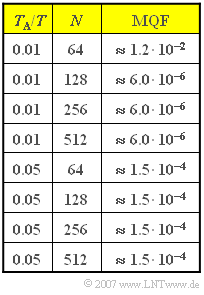# Exercise 5.3Z: Zero-Padding$\rm MQF$ values as a function
of  $T_{\rm A} /T$  and  $N$

We consider the DFT of a rectangular pulse  $x(t)$  of height  $A =1$  and duration  $T$.  Thus the spectral function  $X(f)$  has a  $\sin(f)/f$–shaped course.

For this special case the influence of the DFT parameter  $N$  is to be analysed, whereby the interpolation point distance in the time domain should always be  $T_{\rm A} = 0.01T$  or  $T_{\rm A} = 0.05T$.

The resulting values for the "mean square error"  $\rm (MSE)$  of the grid values in the frequency domain are given opposite for different values of   $N$.  Here, we use instead of  $\rm MSE$  the designation  $\rm MQF$   ⇒   (German:  "Mittlerer Quadratischer Fehler"):

$${\rm MQF} = \frac{1}{N}\cdot \sum_{\mu = 0 }^{N-1} \left|X(\mu \cdot f_{\rm A})-\frac{D(\mu)}{f_{\rm A}}\right|^2 \hspace{0.05cm}.$$

Thus, for  $T_{\rm A}/T = 0.01$ ,  $101$  of the DFT coefficients  $d(ν)$  are always different from zero.

• Of these,   $99$  have the value  $1$  and the two marginal coefficients are each equal to  $0.5$.
• If  $N$  is increased, the DFT coefficient field is filled with zeros.
• This is then referred to as  $\text{zero padding}$.

Hints:

### Questions

1

Which statements can be derived from the given MQF values  $($valid for  $T_{\rm A}/T = 0.01$  and  $N ≥ 128)$?

 The  $\rm MQF$ value here is almost independent of  $N$. The  $\rm MQF$ value is determined by the truncation error. The  $\rm MQF$ value is determined by the aliasing error.

2

Let  $T_{\rm A}/T = 0.01$.  What is the distance  $f_{\rm A}$  of adjacent samples in the frequency domain for  $N = 128$  and  $N = 512$?

 $N = 128$:     $f_{\rm A} \cdot T \ = \$ $N = 512$:     $f_{\rm A} \cdot T \ = \$

3

What does the product  $\text{MQF} \cdot f_{\rm A}$  indicate in terms of DFT quality?

 The product  $\text{MQF} \cdot f_{\rm A}$  considers the accuracy and density of the DFT values. The product  $\text{MQF} \cdot f_{\rm A}$  should be as large as possible.

4

$N = 128$  is now fixed.  Which statements apply to the comparison of the DFT results with  $T_{\rm A}/T = 0.01$  und  $T_{\rm A}/T = 0.05$ ?

 With  $T_{\rm A}/T = 0.05$  you get a finer frequency resolution. With  $T_{\rm A}/T = 0.05$  the  $\rm MQF$ value is smaller. With  $T_{\rm A}/T = 0.05$  the influence of the truncation error decreases. With  $T_{\rm A}/T = 0.05$  the influence of the aliasing error increases.

5

Now  $N = 64$.  Which statements are true for the comparison of the DFT results with  $T_{\rm A}/T = 0.01$  und  $T_{\rm A}/T = 0.05$ ?

 With  $T_{\rm A}/T = 0.05$  you get a finer frequency resolution. With  $T_{\rm A}/T = 0.05$  the  $\rm MQF$ value is smaller. With  $T_{\rm A}/T = 0.05$  the influence of the truncation error decreases. With  $T_{\rm A}/T = 0.05$  the influence of the aliasing error increases.

### Solution

#### Solution

(1)  Proposed solutions 1 and 3 are correct:

• Already with  $N = 128$,  $T_{\rm P} = 1.28 \cdot T$, i.e. larger than the width of the rectangle.
• Thus the truncation error plays no role at all here.
• The  $\rm MQF$ value is determined solely by the aliasing error.
• The numerical values clearly confirm that  $\rm MQF$  is (almost) independent of  $N$.

(2)  From  $T_{\rm A}/T = 0.01$  follows  $f_{\rm P} \cdot T = 100$.

• The supporting values of  $X(f)$ thus lie in the range  $–50 ≤ f \cdot T < +50$.
• For the distance between two samples in the frequency range,   $f_{\rm A} = f_{\rm P}/N$ applies. This gives the following results:
• $N = 128$:   $f_{\rm A} \cdot T \; \underline{\approx 0.780}$,
• $N = 512$:   $f_{\rm A} \cdot T \; \underline{\approx 0.195}$.

(3)  The first statement is correct:

• For  $N = 128$ , the product is  $\text{MQF} \cdot f_{\rm A} \approx 4.7 \cdot 10^{-6}/T$.  For  $N = 512$ , the product is smaller by a factor of about  $4$ .
• This means that „zero padding” does not achieve greater DFT accuracy, but a finer "resolution" of the frequency range.
• The product  $\text{MQF} \cdot f_{\rm A}$  takes this fact into account; it should always be as small as possible.

(4)  Proposed solutions 1 and 4 are correct:

• Because of  $T_{\rm A} \cdot f_{\rm A} \cdot N = 1$ , a constant  $N$  always results in a smaller  $f_{\rm A}$  value when  $T_{\rm A}$  is increased.
• From the table on the information page, one can see that the mean square error  $\rm (MQF)$  is significantly increased  $($by a factor of about  $400)$.
• The effect is due to the aliasing error, since the transition from  $T_{\rm A}/T = 0.01$  auf  $T_{\rm A}/T = 0.05$  reduces the frequency period by a factor of  $5$ .
• The truncation error, on the other hand, continues to play no role with the rectangular pulse as long as  $T_{\rm P} = N \cdot T_{\rm A}$  is greater than the pulse duration  $T$.

(5)  All statements are true:

• With the parameter values  $N = 64$  and  $T_{\rm A}/T = 0.01$ , an extremely large truncation error occurs.
• All time coefficients are  $1$, so the DFT incorrectly interprets a DC signal instead of the rectangular function.